Next: Energy Stored by Capacitors Up: Capacitance Previous: Dielectrics

## Capacitors in Series and in Parallel

Capacitors are one of the standard components in electronic circuits. Moreover, complicated combinations of capacitors often occur in practical circuits. It is, therefore, useful to have a set of rules for finding the equivalent capacitance of some general arrangement of capacitors. It turns out that we can always find the equivalent capacitance by repeated application of two simple rules. These rules related to capacitors connected in series and in parallel.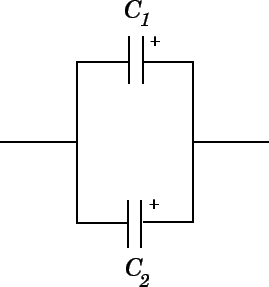Consider two capacitors connected in parallel: i.e., with the positively charged plates connected to a common input'' wire, and the negatively charged plates attached to a common output'' wire--see Fig. 15. What is the equivalent capacitance between the input and output wires? In this case, the potential differenceacross the two capacitors is the same, and is equal to the potential difference between the input and output wires. The total charge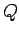, however, stored in the two capacitors is divided between the capacitors, since it must distribute itself such that the voltage across the two is the same. Since the capacitors may have different capacitances,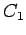and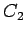, the charges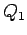and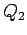may also be different. The equivalent capacitance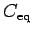of the pair of capacitors is simply the ratio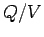, where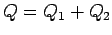is the total stored charge. It follows that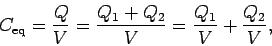(113)

giving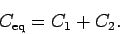(114)

Here, we have made use of the fact that the voltageis common to all three capacitors. Thus, the rule is:
The equivalent capacitance of two capacitors connected in parallel is the sum of the individual capacitances.
Forcapacitors connected in parallel, Eq. (114) generalizes to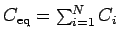.Consider two capacitors connected in series: i.e., in a line such that the positive plate of one is attached to the negative plate of the other--see Fig. 16. In fact, let us suppose that the positive plate of capacitor 1 is connected to the input'' wire, the negative plate of capacitor 1 is connected to the positive plate of capacitor 2, and the negative plate of capacitor 2 is connected to the output'' wire. What is the equivalent capacitance between the input and output wires? In this case, it is important to realize that the chargestored in the two capacitors is the same. This is most easily seen by considering the internal'' plates: i.e., the negative plate of capacitor 1, and the positive plate of capacitor 2. These plates are physically disconnected from the rest of the circuit, so the total charge on them must remain constant. Assuming, as seems reasonable, that these plates carry zero charge when zero potential difference is applied across the two capacitors, it follows that in the presence of a non-zero potential difference the chargeon the positive plate of capacitor 2 must be balanced by an equal and opposite charge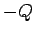on the negative plate of capacitor 1. Since the negative plate of capacitor 1 carries a charge, the positive plate must carry a charge. Likewise, since the positive plate of capacitor 2 carries a charge, the negative plate must carry a charge. The net result is that both capacitors possess the same stored charge. The potential drops,and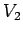, across the two capacitors are, in general, different. However, the sum of these drops equals the total potential dropapplied across the input and output wires: i.e.,. The equivalent capacitance of the pair of capacitors is again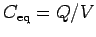. Thus,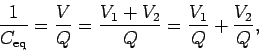(115)

giving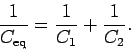(116)

Here, we have made use of the fact that the chargeis common to all three capacitors. Hence, the rule is:
The reciprocal of the equivalent capacitance of two capacitors connected in series is the sum of the reciprocals of the individual capacitances.
Forcapacitors connected in series, Eq. (116) generalizes to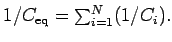Next: Energy Stored by Capacitors Up: Capacitance Previous: Dielectrics
Richard Fitzpatrick 2007-07-14